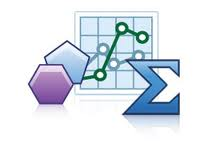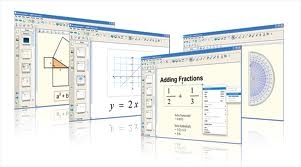• SMART Notebook Math ToolsEducation

Learn the basics on how to use your SMART Notebook Math Tools in the classroom.

Self-paced tutorials

• Drawing with Measurement Tools – tutorial
• Using Math Tools to Measure – tutorial
• Creating Geometric Shapes – tutorial
• Using the Equation Editor – tutorial
• Creating Graphs with SMART Notebook Math Tools – tutorial
• Generating Graphs with Tables and Equations – tutorial
• Using Math Ink – video

Videos

• Introducing Notebook Math Tools – video
• Teaching Secondary Math with SMART Notebook Math Tools – video